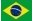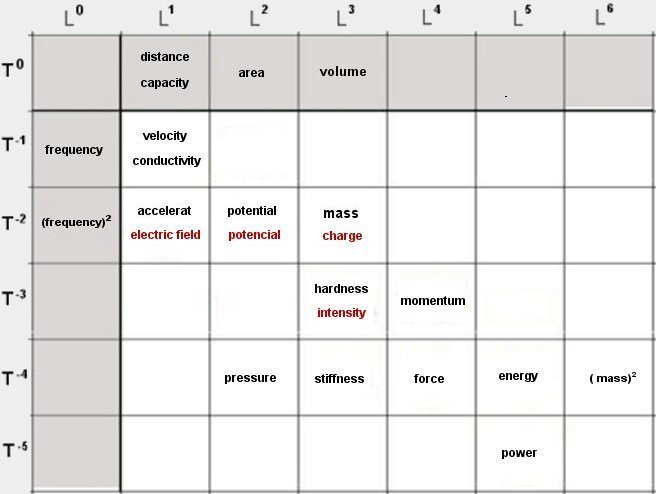Dimensions of an electric chargeWhen writing the formulas of attraction of two masses M (Newton's law) and two charges Q (Coulomb's force), both are oppositely charged forces ( attraction and repulsion)       QQ/R²   =     ( -1 )   MM/R²                  (1)         QQ       =     ( i ² )    MM                        (2)   On each side in equation (1) there is a "force". Both these forces should, according to our inicial hypothesis, have the same "dimensions". This force will be named "negative" if it is an attraction force (two masses that are attracted to each other, according to Newton) and "positive" (when two electric charges of the same signs repel each other, according to Coulomb's formula) . Equation (2) provides two solutions to Q ==>> Q = i M   and   Q = - i M   with  (i)² = - 1 We obtain therefore two types of electric charges that have dimensions: i M and - i M , or i L³/T² and - i L³/T² Those two types of charges will be named "positive charge" and "negative charge". We see immediately that two charges of the same signs repel each other (negative force) and two charges of opposite signs attract each other (positive force). Similarly, two positive or two negative masses will attract each other (negative force) and two oppositely charged masses will repel each other (positive force), according to the same Newton formula ( 1/ R² ). The dimensions of charge will therefore be the same as the dimensions of mass, but complex: i L³/T² or - i L³/T². Some electric quantities will also have "complex" dimensions. i represents a "complex" or "imaginary" number, defined by the relation: ( i)² = - 1 We can even assume that a "space-time" deformation will have a real component (mass) and a complex component (charge). In a general M + iQ and the general formula of attraction of "masses-charges" becomes: F = ((M1+iQ1).(M2+iQ2))/R²     (3) This force will have two real components (two masses attract each other and two charges of the same signs repel each other). ((M1).(M2))/R²   and   ((iQ1).(iQ2))/R²   This same force will have two complex components ( charge does not affect mass). ((M1).(iQ2))/R²   and   ((M2).(iQ1))/R² In Lorentz–Heaviside units (also called rationalized) the Coulomb constant is   ke = 1/4 p )Complex quantities are in red Since we know that the electric charge has i L3 T -2 we can determine the dimensions of: Intensity I (ampere) = coulomb / second or i L3 T -2 / T = i. L3 T -3 Potential ( volt ) = coulomb / metre or i L3 T -2 / L = . i L2 T -2 Electric field = volt / metre or i L2 T -2 / L = . i L T -2 Those same quantities can vary in relation to time: Variation of intensity in relation to time, or i L3 T -3 / T = i L3 T -4 Variation of potential in relation to time, or i L2 T -2 / T = i L2 T -3 Variation of electric field in relation to time, or i L T -2 / T = i L T -3 Electrical quantities (charge, potential, intensity, electric field) would be therefore complex quantities, contrary to mechanical quantities, that would be real and directly measurable. To measure an electrical quantity (charge, potential, intensity, electric field) it will be necessary to use electricity to obtain a real, measurable quantity (for example force or displacement). An electric charge will produce a force (directly measurable) when interacting with another charge or with an electric field. When combining two complex quantities (i² = -1) we return to the real and measurable. For example, a charge (complex quantity) situated in an electric field (another complex quantity) will result in a force (real quantity) that will have the same dimensions as mechanical force ( L4 T -4 ). We can also see that an electric potential (volt V) with a certain intensity (ampere A ) will result in a power ( W = VA ) that will have the same dimensions as a mechanical power ( L5 T -5 ). On the other hand, a charge (complex quantity) situated in a gravitational field (real quantity) will result in an unmeasurable real quantity (complex force?). Some fundamental relations in physics (complex quantities are in red, " i L3 T-2" will be written as " L3 T -2 ") Q.Ê = F      ( L3 T -2 ) ( L1 T -2 )  =   ( L4 T -4 )    =>    charge . elecric field = force Q.V = E      ( L3 T -2 ) ( L² T -2 )   =   ( L5 T -4 )    =>    charge . potencial = energy Q / R = V    ( L3 T -2 ) ( L -1)  =   ( L2 T -2 )    =>    charge / distance = potencial Q / R² = Ê    ( L3 T -2 ) ( L-2 )  =  ( L1 T -2 )    =>    charge / (distance)² = electric field Q² / R = E     ( L3 T -2 )² / (L-1)  =  ( L5 T -4 )    =>    (charge)² / distance = (charge)² / capacity= energy Q² / R² = F    ( L3 T -2 )² / ( L-2 ) =  ( L4 T -4 )    =>    (charge)² / (distance)² = forçe V.I = W        ( L2 T -2 ) ( L3 T -3 )  =  ( L5 T -5 )    =>    potencial . intensity = power I / V = 1 / R   ( L3 T -3 ) ( L-2 T 2 )  = ( L1 T -1 )    =>    intensity / potencial = conductivity = 1/ resistivity V.V = F         ( L2 T -2 ) ( L2 T- 2 ) = ( L4 T -4  ) =>    potencial . potencial = force ( I /T ) S = V   ( L3 T-4 ) ( L-1 T 2 ) = ( L2 T -2 )    =>    (intensity variation).(self-induct coeficient) = potencial C V = Q       ( L1 T 0 )( L2 T -2 ) = ( L3 T -2 )    =>    capacity. potencial = charge I² . R = W     ( L3 T -3 )² (L-1 T 1) = ( L5 T -5 )    =>    (intensity)² . resistivity) = power   Dimensions of some quantities in physics (complex quantities in red) acceleration = ( L1 T -2 ) capacity= ( L1 T 0 ) = space or distance electric field ( L1 T -2 ) ( Volts / meter ) gravitational field ( L1 T -2 ) charge ( L3 T -2 ) ( Coulomb ) conductivity ( L1 T -1 )  =  1 / resistivity  ( speed ) hardness( L3 T -3 ) energy ( L5 T -4 ) = work ( Joule ) force ( L4 T -4 ) ( Newton ) inductance ( L-1 T 2 ) = 1 / acceleration   ( Henry ) intensidade( L3 T -3 ) ( Ampère ) momentum ( L4 T -3 ) mass ( L3 T -2 ) electric potential ( L2 T -2 ) ( Volt ) gravitational potential ( L2 T -2 ) pressure ( L2 T -4 ) ( Bar ) power ( L5 T -5 ) ( Watts ) resistivity ( L-1 T 1 ) = 1 / speed hardness( L3 T -4 ) speed ( L1 T -1 )

 Space - Time Appendix 1 Appendix 2